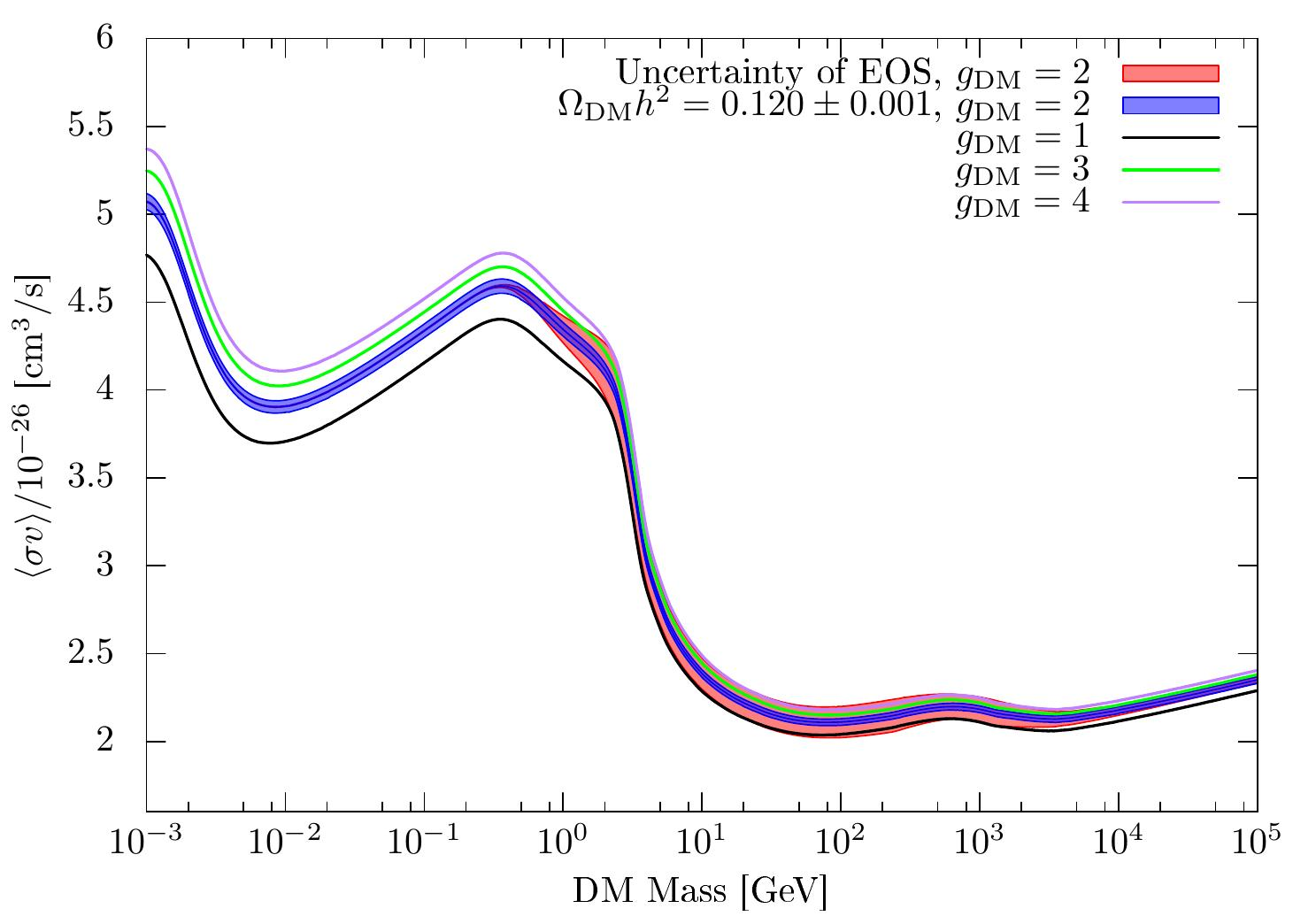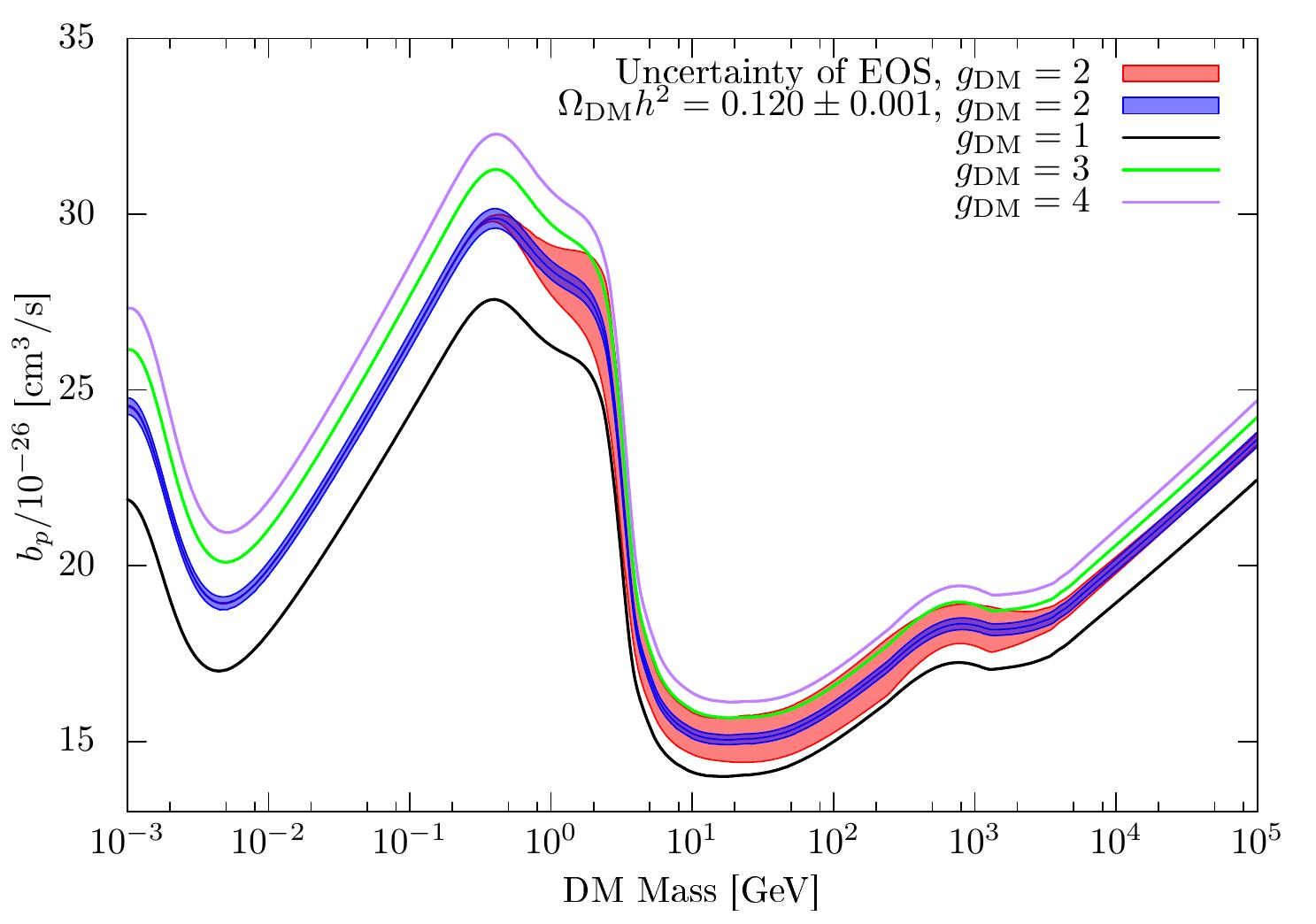top

## Equation of State and WIMP Dark Matter

Tabulated data of the effective degrees of freedom and the dark matter cross section of this paper are distributed.
You can get the data of the effective degrees of freedom here.
The data format is:
Temperature [GeV], g_{s}, g_{\rho}
This data file is directly available in the Program Micromegas v_5.
In order to use the present estimation, simply put
in the main program.
Though we esimate g_{s}(T_0) = 3.931, the Micromegas seems to adopt g_{s}(0) = 3.910 when converting Y_{DM} to Omega h^2.
Therefore multiplying factor 1.005 by the Micromegas output with EOS2020.dat reproduces well our estimation.
There are several uncertainties of the estimation of the degrees of freedom.
We also provide the data for the upper error and lower error.

We also apply it to simplified WIMP dark matter models.
We estimate the DM annihilation cross section to realize the current observation $\Omega h^2 = 0.120 \pm 0.001.$
S-wave
real scalar, complex scalar or Majorana fermion, vector, Dirac fermion
P-wave
real scalar, complex scalar or Majorana fermion, vector, Dirac fermion
The data format is:
DM Mass [GeV], sigma v (a_s or b_p) [cm^3/s /10^{-26}], error from DM abudance mesurement, error from EOS

### Equation of State

The effective degrees of freedom are defined as: $g_{\rho}(T) \equiv \frac{\rho(T)}{\left[\frac{\pi^2 T^4}{30}\right]},\quad g_{s}(T) \equiv \frac{s(T)}{\left[\frac{2\pi^2 T^3}{45}\right]}.$Effective degrees of freedom and its uncertainty. The data: central curve, upper curve, lower curve.

### WIMP Cross Section

The Boltzmann equation of the WIMP abundance is given by $\frac{d n_{\rm DM}}{dt} + 3 H n_{\rm DM} = -\langle \sigma v \rangle (n_{\rm DM}^2 - n_{\rm DM,eq}^2 ).$ The annihilation cross section is parameterized as: $\sigma v = a_s + b_p v^2 + \cdots,$ or $\langle \sigma v \rangle = a_s + b_p \frac{6 T}{m_{\rm DM}} + \cdots.$S-wave dominated case.P-wave dominated case.